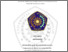## KAJIAN MATRIKS-M NON SINGULAR PADA PENJUMLAHAN SUBDIRECT (LANGSUNG-SEBAGIAN)

NUR DIANA, FITRIYAH (2009) KAJIAN MATRIKS-M NON SINGULAR PADA PENJUMLAHAN SUBDIRECT (LANGSUNG-SEBAGIAN). Other thesis, University of Muhammadiyah Malang.Preview
Text
KAJIAN_MATRIKS.pdf

## Abstract

Linear algebra is represent one of the branch in mathematics science, such as matrix algebra. The addition matrix are represent one of them. And also, there are extension of addition matrix which sub direct is represent extension of addition matrix. There also some special matrix which represent the differential of previous matrix which one of them called M-matrix. This thesis will present M-matrix that having some benefit. For example : in calculation of convergent iteration sum method to solve large equation system, and can used in area like input and output system at economic model, queue theory, engineering and others. The purpose of this thesis is to describe and prove theorem of some properties from sub direct sums of nonsingular M-matrices Some properties from sub direct sums of nonsingular M-matrices are not commutative; the result of its sum is nonsingular if the inverse from sum sub direct is nonsingular, the result of sub direct sums of nonsingular M-matrices is nonsingular if the element of each blocks matrix from inverse is nonnegative, the result of sub direct lower and upper triangular sums of nonsingular M-matrices is nonsingular M-matrices, inverse from nonsingular M-matrices is nonsingular if the sub direct sum is nonsingular, inverse from sum inverse nonsingular matrix-M is nonsingular M-matrix if inverse from sum inverse of nonsingular matrix is Z-matrix. The result of sub direct sums of nonsingular M-matrices is nonsingular M-matrices.

Item Type: Thesis (Other) L Education > L Education (General) Faculty of Teacher Training and Education > Department of Mathematics Education (84202) Anggit Aldila 21 Jun 2012 03:25 21 Jun 2012 03:25 http://eprints.umm.ac.id/id/eprint/8874View Item